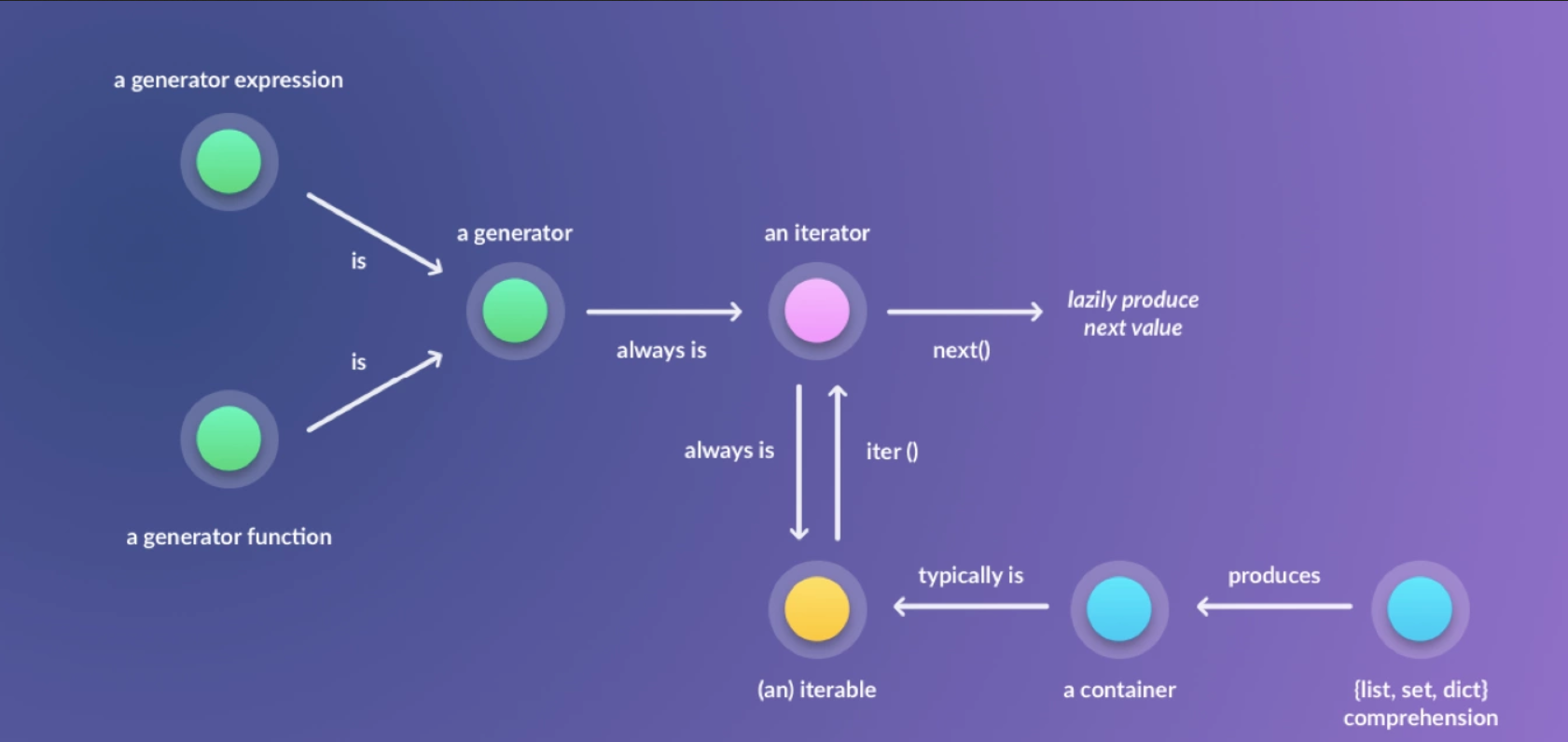# 【Python】解析Python中的迭代器

contents structure [-]# 1. Iterator VS Iterable

## 可迭代对象(Iterable)

a_set = {1, 2, 3}#定义set数据类型，set是可迭代类型
b_iterator = iter(a_set)#得到set的迭代器
#Output: 1
print(next(b_iterator))

#Output: <class 'set'>
print(type(a_set))

#Output: <class 'set_iterator'>
print(type(b_iterator))

class Series(object):
def __init__(self, low, high):
self.current = low
self.high = high

def __iter__(self):
return self

def __next__(self):
if self.current > self.high:
raise StopIteration
else:
self.current += 1
return self.current - 1

n_list = Series(1,10)
print(list(n_list))

# 2.Itertools 模块

Itertools是Python的内置模块，其中包含了能够创建迭代器的函数。简而言之，它提供了许多能够与迭代器交互的方法。

from itertools import count
sequence = count(start=0, step=1)
while(next(sequence) <= 10):
print(next(sequence),end=" ")

1 3 5 7 9 11

Itertools中的cycle函数可以创建无限迭代器，例如：

from itertools import cycle
dessert = cycle(['Icecream','Cake'])
count = 0
while(count != 4):
print('Q. What do we have for dessert? A: ' + next(dessert))
count+=1

Q. What do we have for dessert? A: Icecream
Q. What do we have for dessert? A: Cake
Q. What do we have for dessert? A: Icecream
Q. What do we have for dessert? A: Cake

# 3.生成器（Generator）

def series_generator(low, high):
while low <= high:
yield low
low += 1

n_list = []
for num in series_generator(1,10):
n_list.append(num)

print(n_list)

def test():
print("进入test函数")
for i in range(2):
print("yield number ",i)
yield i
if "__main__" == __name__:
print("开始调用test")
res =  test()
print("结束调用test")
next(res)
next(res)

开始调用test

yield number 0

yield number  1

python中生成器可以分为生成器函数和生成器表达式，这两种类型的表现形式完全不同。

squares = (x * x for x in range(1,10))
print(type(squares))
print(list(squares))

<class 'generator'>
[1, 4, 9, 16, 25, 36, 49, 64, 81]

https://www.datacamp.com/community/tutorials/python-iterator-tutorial

posted @ 2019-06-03 23:59  HDWK  阅读(...)  评论(...编辑  收藏